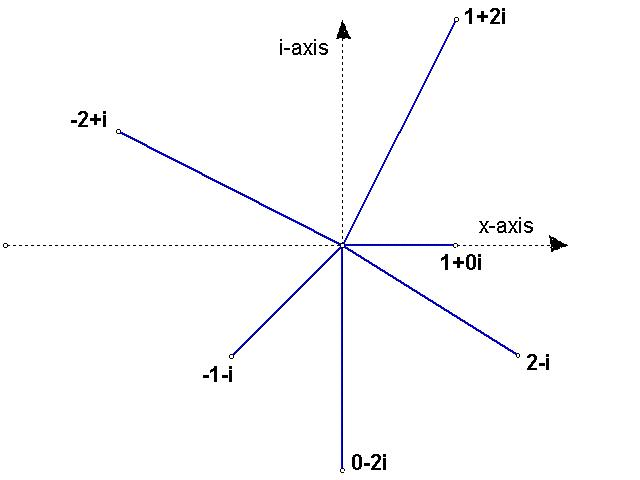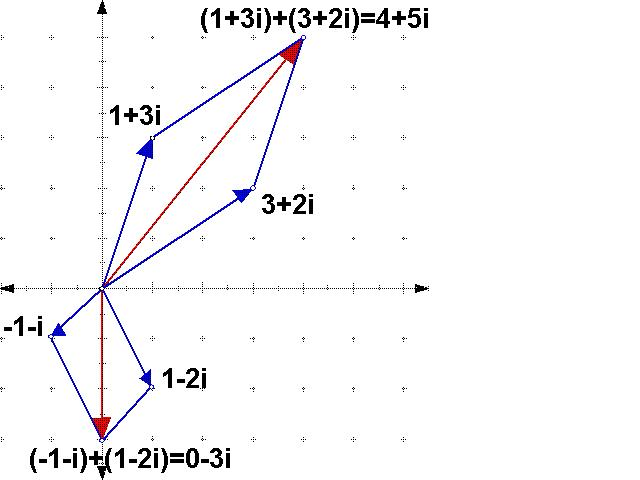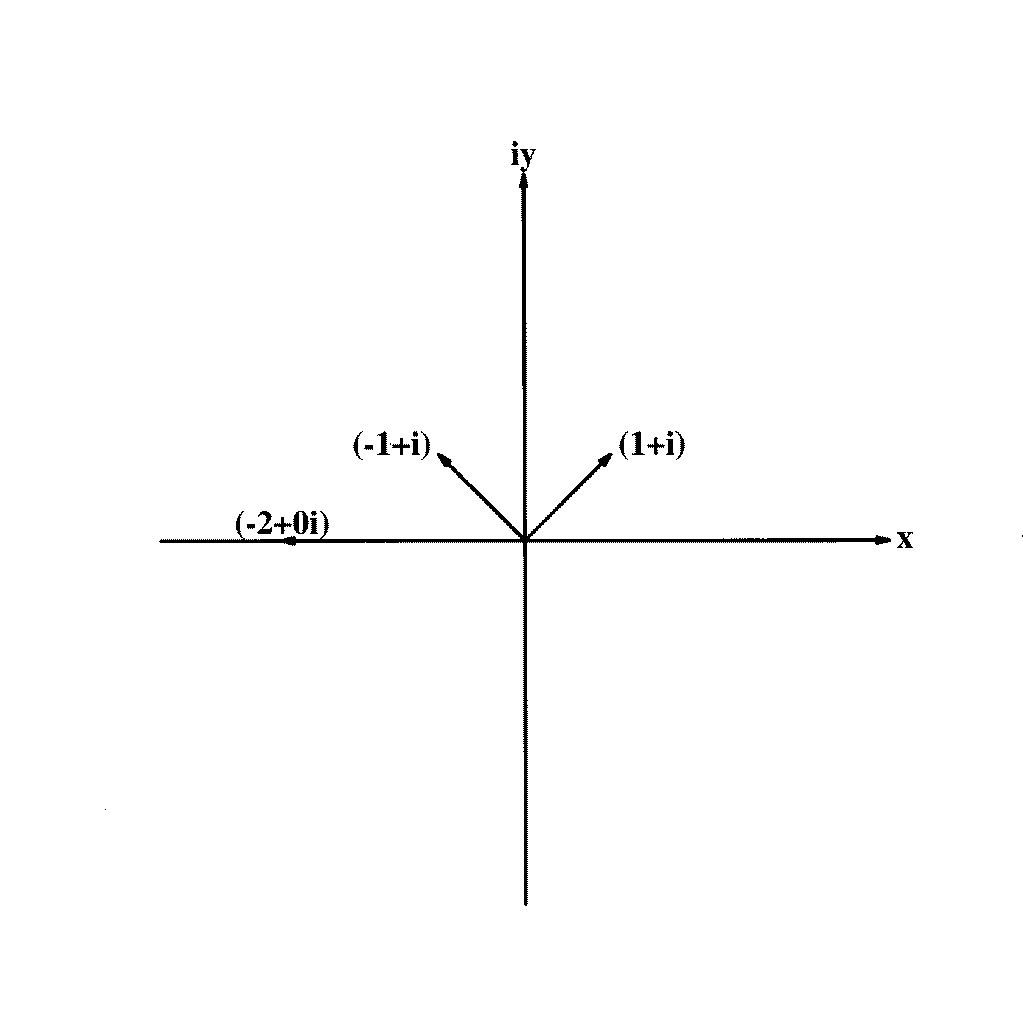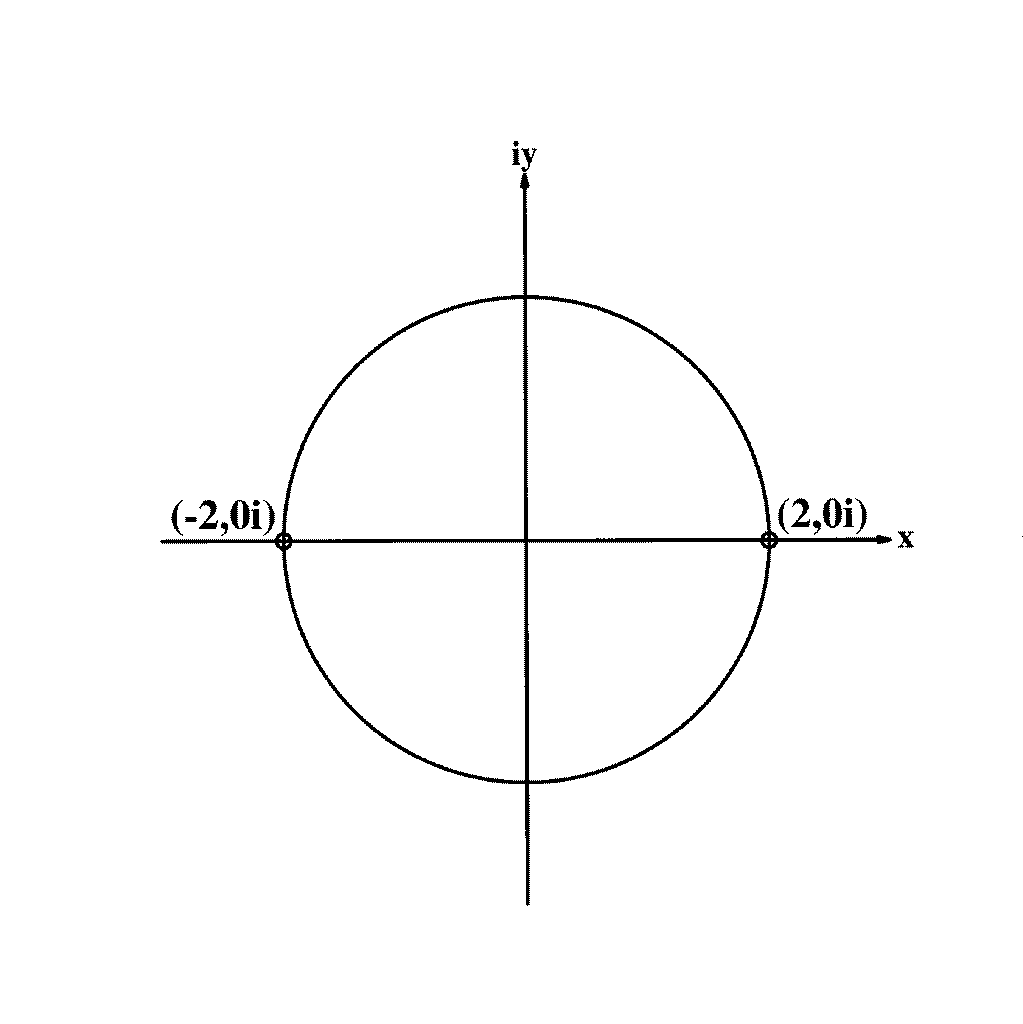# COMPLEX NUMBERS: "i" LOVE 'EM!

Complex numbers show up in a variety of interesting, real-world applications, such as the analysis of electronic circuits, high-speed flow over a jet plane, and so on. They are also heart and soul of certain fractals, such as that recently-discovered, four-dimensional object of nature represented by the union of the Mandelbrot and Julia sets.

This site introduces the basic idea behind complex numbers: where do they come from, how does one add them and so on? If you are already familiar with complex numbers, you may wish to skip ahead to those sections you would like to brush up on:

## I. BASIC DEFINITIONS

In mathematics, as in life, we often run up against a wall where something just seems impossible or unreal. In mathematics, however, working with the "unreal" often produces very real results. Complex numbers are an excellent example of this phenomenon.

The square root of negative one seems impossible, unreal. But it is actually very real, and a crucially important number in mathematics. So, we give it a name: i.

• THE IMAGINARY UNIT: Let i = the imaginary unit, defined such that i 2 = -1.

• COMPLEX NUMBERS: Have both a real part and an imaginary part. Examples:
• 3+6i (3 is the real part, 6i is the imaginary part)
• 4-2i B
• x+yi

• REAL NUMBERS: When the imaginary part of a complex number is zero, you get a real number! Examples:
• 5
• 87
• square root of 97.3

• PURELY IMAGINARY NUMBERS: These are complex numbers whose real part is zero. Examples:
• 5i
• -2.75i

• AMPLITUDE AND MODULUS OF COMPLEX NUMBERS: These will be easier to explain in the next section on representing complex numbers graphically in the complex plane.
Forward
Back to Beginning
Back to Definitions Page

## II. REPRESENTING COMPLEX NUMBERS IN THE COMPLEX PLANE

Graphing complex numbers is done in a manner very similar to plotting a point (x, y) in rectangular coordinates; the main difference is that the Y-axis now represents IMAGINARY numbers, rather than the REALS, as when plotting points in rectangular coordinates.  Below is a graph demonstrating some numbers (points) in the complex plane:Incidentally, from this figure we can define the amplitude (or norm) and the modulus of a complex number. The amplitude is the length of the "arrow" or line which connects the complex nummber to the origin. The amplitude of 1+2i, for example, is (by the Pythagorean Theorem) the square root of 12 + 22, which is the sqaure root of five. The modulus is the angle that the "arrow makes" when measured from the x-axis; 1+0i, for example, has a modulus of zero degrees, and -1-i has a modulus of 135 degrees. You can find these angles using the trigonometric identity that theta = inverse tangent of (i-component) over (x-component) - more examples can be found in the last section on finding the nth roots of any number.

Forward
Backward
Back to Beginning
Back to Definitions Page

• (3+2i) + (1+3i) = 4+5i
• (-1-i) + (1-2i) = 0-3i

Here's how that looks in the complex plane:Forward
Backward
Back to Beginning
Back to Definitions Page

## SUBTRACTING COMPLEX NUMBERS

A To subtract complex numbers, we subtract the real parts and the imaginary parts. Examples:

• (3+2i) - (1+3i) = (3-1)+i(2-3) = 2-i
• (-2-i) - (2+3i) = (-2-2)+i(-1-3) = -4-4i

Here's how that looks in the complex plane - note that it's the same as adding, except first we have to turn around backwards the "arrow" that we're subtracting:Forward
Backward
Back to Beginning
Back to Definitions Page

## MULTIPLYING COMPLEX NUMBERS

Well, there's an algebraic way to do this and a pictorial way to do this. The algebraic method uses the good old "FOIL" method (multiply the first terms, then the outer terms, then the inner terms, then the last terms). B The key is to remember that i2=-1. Examples:

• (1+i)*(2-i) = 1*2 + 1*(-i) + i*2 + i*-i = 2-i+2i-i2 = 2-i+2i+1 = 3+i
• (1+i)*(-1+i) = -1 + i - i + i2 = -1 -1 = -2

Showing what happens in the complex plane is rather more work, but I'm sure you'll agree that the beauty of it is definitely worth the effort!

Basically, it involves three steps:

1. Draw your complex numbers as arrows, then refer to your trig notes (or the formulas below) to find the length of each arrow and the angle from each to the x-axis. This is the "hard part."
2. To get the length of the answer, multiply the lengths of your little A arrows! (Recall that that's called the norm or the amplitude)
3. To see which way the answer-arrow points, add the angles of your little arrows! Recall that that's called the modulus)

Now, as Bill Nye would say, isn't that wild? Let's do an example. Let's show that:

(1+i)*(-1+i)=-2:Here's the step-by-step:

1. Let x be the real component and y the imaginary component of an arrow. We get the length of the arrow from the Pythagorean theorem: r=(x2 + y2)0.5. So, the length of (1+i) is (12 + 12)0.5 = 20.5 and the length of (-1+i) is ((-1)^2 + 1^2)^1/2 = 2^1/2 . B
2. To get the angle that (1+i) makes with the x-axis, take the inverse tangent of y (=1) over x (=1) and you get 45 degrees. Similarly, (-1+i) is found to be at 135 degrees from the x-axis.
3. So the "answer" has a length equal to 2^1/2 * 2^1/2 = 2!
4. And the "answer" is at 180 degrees, which is 45 degrees plus 135 degrees!

So what we have here is a stretching of the arrows and a rotation in the complex plane. Neat, eh? By the way, notice that we've just verified graphically the result we got earlier using the FOIL method!

A Forward
Backward
Back to Beginning
Back to Definitions Page

# EXPONENTIATION OF COMPLEX POWERS

We'll be really brief and to the point here. Let's say you have z = 5+2i and you want to find z2. Remember how exponents work? The superscript of two tells you to multiply z by itself two times. So, you need to multiply (5+2i)*(5+2i). Refer to the previous section on multiplying complex numbers if you're not sure how to do that; if you try it yourself you should be able to verify that the answer is 25 + 20i + 4i2 = 21 + 20i.
Forward
Backward
Back to Beginning
Back to Definitions Page

# FINDING THE NTH ROOTS OF ANY NUMBER IN THE WORLD

First of all, here's a nifty rule of thumb you can remember for all time: No matter what number you're talking about, if you're taking it's nth root, there are n of them! That means every number has two square roots, three cube roots, ninety-seven ninety-seventh roots, and so on. No matter what.

Ok, so how do we go about finding those roots? Again, it's a three-step process. See if this makes sense to you:

1. Plot your number that you want to take the root of (x+iy), draw its arrow, and work out its length and the angle it makes to the x-axis. B Remember: A r = (x^2 + y^2)^1/2 and theta = tan^-1 y/x .
2. The first root has length equal to the "regular" nth root of r, and direction theta / n.
3. The other n roots are equally spaced on the circle of radius equal to the nth root of r and centered at the origin!
EXAMPLES OF ROOT CIRCLES

To show you how this works, five rather nipziffic examples have been worked out. First, the square roots of four are shown below. After taking a gander at those, there are four A more examples you can click on and check out - but before you do, you may want to have a little fun and see if you can work them out in your mind (or even on paper) before you actually look at the "answers"!

## WELCOME TO THE SQUARE ROOTS OF FOUR!# HERE'S HOW YOU FIND THEM:

• (4+0i) has length or magnitude 4 and an angle to the x-axis, or modulus, of zero degrees;
• so the root circle has radius 41/2 = 2, A
• the first root is at theta1 = 0/2 = 0 degrees,
• and the remaining root is at theta = 0 + 360/2 = 180 degrees. A

## MORE ROOTS YOU CAN CHECK OUT!

• Now that you've seen the square roots of four, what do you suppose the square roots of 4i would look like? Click here to find out!
• What? The number 8 has two more cube roots besides 2? I want to see them!
• Ok, so where would the fifth roots of 8 be? Click on this to find out!
• One more: Let's just make up a number, say, 15+15i. Where, oh where, would we find all five of its fifth roots? Click here A and it shall be made known to you!

Backward
Back to Beginning
Back to Definitions Page